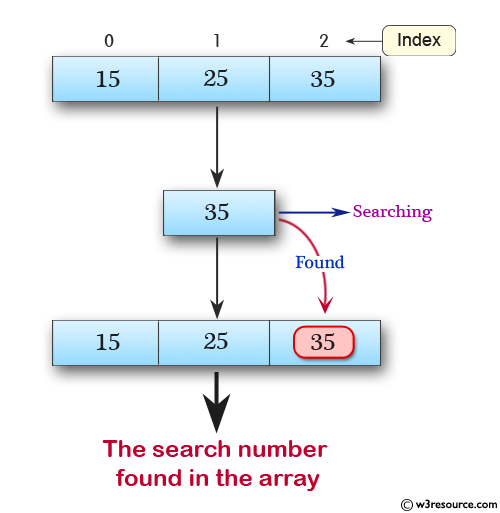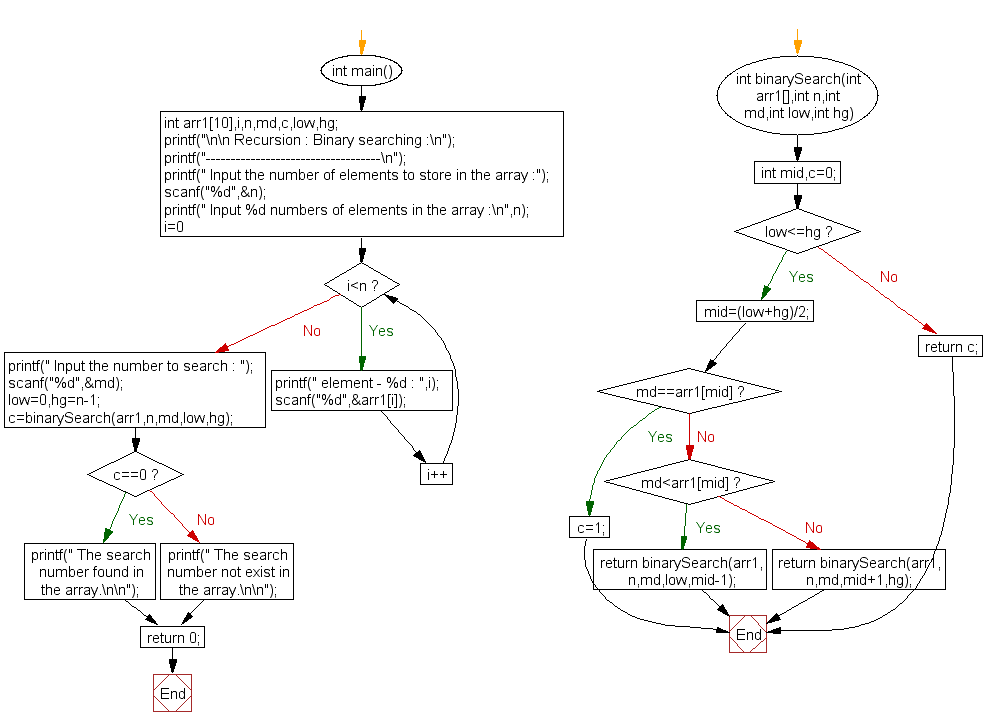﻿ C Program: Binary searching - w3resource

# C Exercises: Binary searching

## C Recursion : Exercise-21 with Solution

Write a program in C for binary search using recursion.

Pictorial Presentation:Sample Solution:

C Code:

``````#include <stdio.h>
int binarySearch(int*, int, int, int, int);

int main()
{
int arr1, i, n, md, c, low, hg;

printf("\n\n Recursion : Binary searching :\n");
printf("-----------------------------------\n");
printf(" Input the number of elements to store in the array :");
scanf("%d", &n);
printf(" Input %d numbers of elements in the array in ascending order :\n", n);
for (i = 0; i < n; i++)
{
printf(" element - %d : ", i);
scanf("%d", &arr1[i]);
}
printf(" Input the number to search : ");
scanf("%d", &md);
low = 0, hg = n - 1;
c = binarySearch(arr1, n, md, low, hg);
if (c == 0)
printf(" The search number not exists in the array.\n\n");
else
printf(" The search number found in the array.\n\n");
return 0;
}

int binarySearch(int arr1[], int n, int md, int low, int hg)
{
int mid, c = 0;
if (low <= hg)
{
mid = (low + hg) / 2;
if (md == arr1[mid])
{
c = 1;
}
else if (md < arr1[mid])
{
return binarySearch(arr1, n, md, low, mid - 1);
}
else
return binarySearch(arr1, n, md, mid + 1, hg);
}
else
return c;
}
```
```

Sample Output:

``` Input the number of elements to store in the array :3
Input 3 numbers of elements in the array in ascending order :
element - 0 : 15
element - 1 : 25
element - 2 : 35
Input the number to search : 35
The search number found in the array.
```

Explanation:

```int binarySearch(int arr1[], int n, int md, int low, int hg)
{
int mid, c = 0;
if (low <= hg)
{
mid = (low + hg) / 2;
if (md == arr1[mid])
{
c = 1;
}
else if (md < arr1[mid])
{
return binarySearch(arr1, n, md, low, mid - 1);
}
else
return binarySearch(arr1, n, md, mid + 1, hg);
}
else
return c;
}
```

The function binarySearch () takes four arguments: the integer array arr1, the size of the array n, the element to search for md, and the lower and upper bounds of the search low and hg.

• Inside the function, we first compute the middle index mid of the current search range.
• We then compare the element at index mid with the target element md. If they are equal, we set a flag variable c to 1, indicating that we have found the element.
• If the element at index mid is greater than the target element md, we recursively call the function on the lower half of the search range, i.e., from low to mid-1.
• If the element at index mid is less than the target element md, we recursively call the function on the upper half of the search range, i.e., from mid+1 to hg.
• If the lower bound low is greater than the upper bound hg, we have exhausted the search range without finding the element, so we return the flag variable c, which is still set to 0.

Time complexity and space complexity:

The time complexity of the binary search algorithm is O(log n), where n is the size of the array.

The space complexity of the recursive function is O(log n) due to the call stack.

Flowchart:C Programming Code Editor:

Have another way to solve this solution? Contribute your code (and comments) through Disqus.

What is the difficulty level of this exercise?

Test your Programming skills with w3resource's quiz.

﻿

## C Programming: Tips of the Day

__FILE__ macro shows full path

```#include <string.h>

#define __FILENAME__ (strrchr(__FILE__, '/') ? strrchr(__FILE__, '/') + 1 : __FILE__)
For Windows use '\\' instead of '/'.
```

Ref : https://bit.ly/3iEWRoT

We are closing our Disqus commenting system for some maintenanace issues. You may write to us at reach[at]yahoo[dot]com or visit us at Facebook